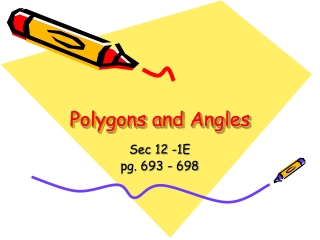DownloadDownload PresentationPolygons and Angles

# Polygons and Angles

Télécharger la présentation## Polygons and Angles

- - - - - - - - - - - - - - - - - - - - - - - - - - - E N D - - - - - - - - - - - - - - - - - - - - - - - - - - -
##### Presentation Transcript

1. Polygons and Angles Sec 12 -1E pg. 693 - 698

2. Definition • Polygon – a simple, closed figure formed by three or more straight line segments. • A polygon is named by the letters of its vertices, written in consecutive order. Not Polygons Polygons

3. Polygons – or Not? • Polygons have • Line segments are called sides • Sides meet only at their endpoints • Points of intersection are called vertices • Not Polygons • Figures have sides that cross each other • Figures are open • Figures have curved sides. Not Polygons Polygons

4. Regular Polygons • An equilateral polygon has all sides congruent. • A polygon is equiangular if all of its angles are congruent. • A regular polygon is equilateral and equiangular with all sides and all angles congruent.

5. Number of Sides 3 4 5 6 7 8 9 10 12 n sides Name Triangle Quadrilateral Pentagon Hexagon Heptagon Octagon Nonagon Decagon Dodecagon n-gon Names of Polygons An 11 sided polygon is sometimes referred to as a undecagon or a hendecagon

6. Interior Angle Sum of a Polygon • The sum of the measures of the angles of a polygon is given by • S = 180 (n – 2) • Where n represents the number of sides. The sum of the interior angles is 540 n = 5 S = 180 (n – 2) S = 180 (5 – 2) = 180 (3) S = 540

7. Work this problem Find the sum of the measures of the interior angles of this polygon. This is a hexagon, so it has 6 sides. S = 180 (n – 2) What is the measure of an individual interior angle? S = 180 (6 – 2) S = 180 ( 4) The measure of an individual interior angle is 720/6 = 120 S = 720